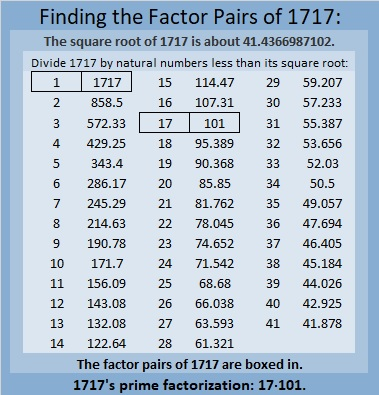# 1717 Factor Fits for Your Valentine

### Today’s Puzzle:

Solve this Valentine-themed Factor Fits puzzle with both logic and heart!### Factors of 1717:

• 1717 is a composite number.
• Prime factorization: 1717 = 17 × 101.
• 1717 has no exponents greater than 1 in its prime factorization, so √1717 cannot be simplified.
• The exponents in the prime factorization are 1 and 1. Adding one to each exponent and multiplying we get (1 + 1)(1 + 1) = 2 × 2 = 4. Therefore 1717 has exactly 4 factors.
• The factors of 1717 are outlined with their factor pair partners in the graphic below.### More About the Number 1717:

1717 is the sum of two squares two different ways:
41² + 6² = 1717, and
39² + 14² = 1717.

1717 is the hypotenuse of FOUR Pythagorean triples:
340 1683 1717, which is 17 times (20-99-101),
492 1645 1717, calculated from 2(41)(6), 41² – 6², 41² + 6²,
808 1515 1717, which is (8-15-17) times 101, and
1092 1325 1717, calculated from 2(39)(14), 39² – 14², 39² + 14².